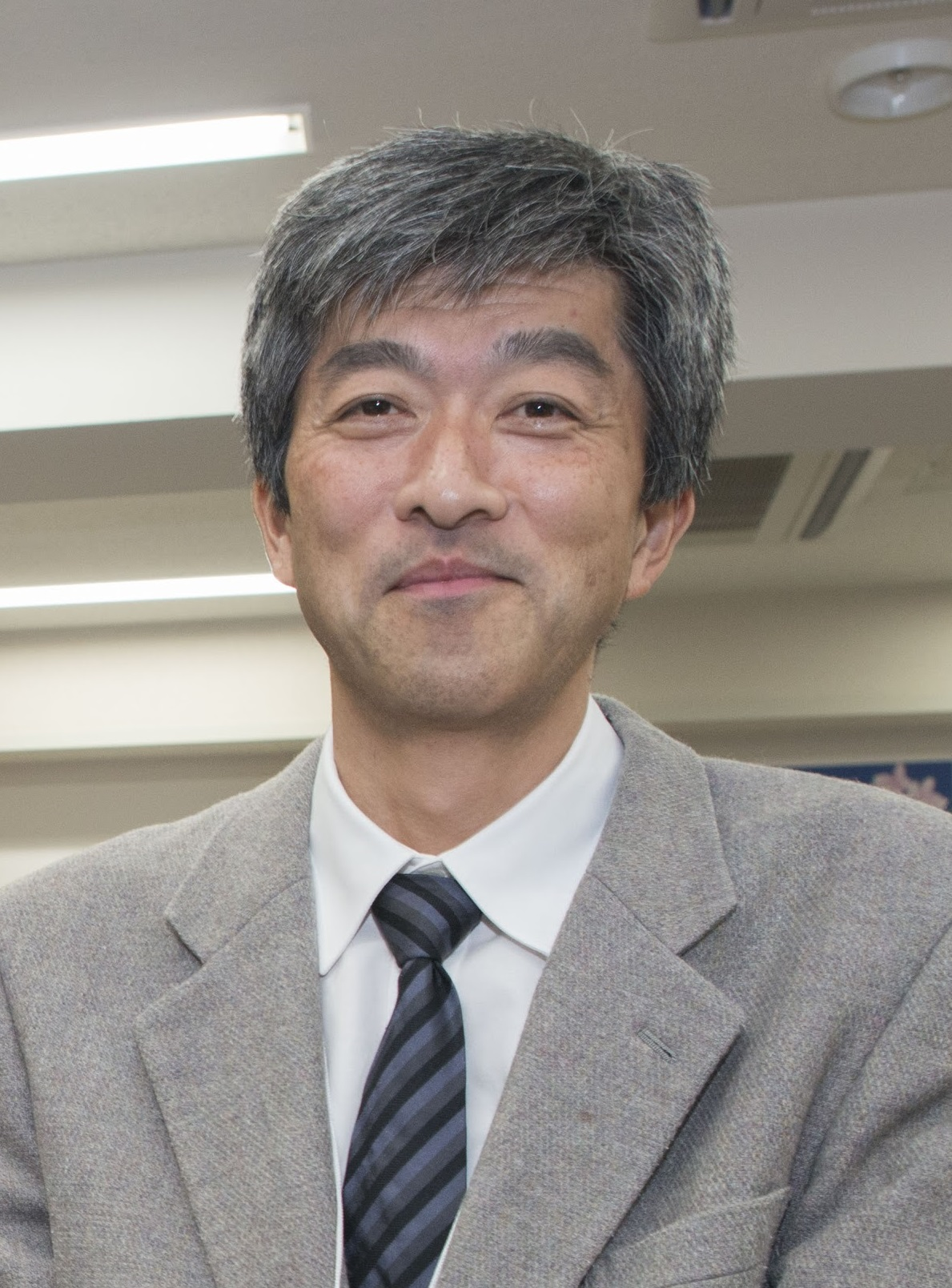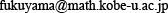Katusi FUKUYAMA Katusi FUKUYAMA Department of Mathematics, Graduate School of Science, Kobe University Division : Applied Mathematics Professor Building X, Room 201Research Field : Theory of lacunary series, Uniform distribution theory, and Probability theory Research Summary : Randomness appears in many forms among researches on lacunary series and uniform distribution theory of numbers. We focus on stochastic properties of lacunary sequences, especially geometric progression, and prove metric discrepancy results. Primary Publications : K. Fukuyama, The central limit theorem for Riesz-Raikov sums, Probability Theory and Related Fields, vol. 100, 57-75, 1994. K. Fukuyama, Almost sure invariance principles for lacunary trigonometric series, Comptes Rendus de l'Academie des Sciences, Paris, series I, vol. 332, 685-690, 2001. K. Fukuyama, The law of the iterated logarithm for discrepancies of $\{\theta^n x\}$, Acta Mathematica Hungarica, vol. 118, 155-170, 2008. K. Fukuyama, A central limit theorem for trigonometric series with bounded gaps, Probability Theory and Related Fields, vol. 149, 139-148, 2011. K. Fukuyama, Metric discrepancy results for alternating geometric progressions, Monatshefte fuer Mathematik, vol. 171, 33-63, 2013. K. Fukuyama, A metric discrepancy result for the sequence of powers of minus two, Indagationes Mathematicae, vol. 25, 487-504, 2014.